# The bath

The bath has water with a volume of 220 l and a temperature of 65°C. Determine the mass m2 of water at 15°C to be poured so that the resulting temperature of the water in the bath is 40°C. We neglect the heat exchange between water and the environment. The mass heat capacity of water is 4200 J/kg ∙ °C.

m2 =  220 l

### Step-by-step explanation: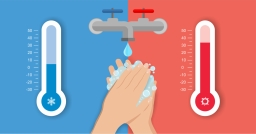Did you find an error or inaccuracy? Feel free to write us. Thank you!Tips to related online calculators
Tip: Our volume units converter will help you with the conversion of volume units.

## Related math problems and questions:

• The bathDad poured 50 liters of water at 60°C into the tub. How much must cold water be added at 10°C to obtain a bath that has a temperature of 40°C?
• Water temperaturesTo 25 liters of water at 50 °C we add 15 liters of water at a different temperature. How many °C should the water be colder than 50 °C so that 40 liters of the water obtained has a temperature 42.5 °C?
• The aluminumThe aluminum object with a weight of 0.80 kg and a temperature of 250°C was placed in the water with a weight of 1.6 kg and a temperature of 23°C. What is the system temperature after reaching equilibrium? We assume that the heat exchange occurred only be
• Heat transferWe placed a lead object weighing 0.4 kg and 250°C in 0.4 L water. What was the initial temperature of water t2 if the object's temperature and the water after reaching equilibrium was 35°C? We assume that the heat exchange occurred only between the lead o
• Water mixingWe have 520 ml of hot water and 640 ml of water at 48°C. What is the temperature of approximately hot water when the resulting mixture has a temperature of 65°C?
• ThereThere is 5 l of water with a temperature of 20°C in the bucket. We add 3 liters of hot water. The resulting temperature will be 30°C. What was the temperature of the hot water?
• Latent heat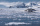How much heat is needed to take from 100 g of water at 20°C to cool to ice at -18°C? Mass heat capacity c (ice) = 21kJ/kg / °C; c (water) = 4.19 KJ/kg / °C and the mass group heat of solidification of water is l = 334kJ/kg
• Ice to boiling water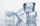Calculate the heat needed to convert 5kg of ice with a temperature of -5°C to water with a temperature of 100°C. c (water) = 4200 J. Kg-1. kg-1, c (ice) = 2100 J. Kg-1. kg-1. the mass group heat of solidification of water is l = 334kJ/kg. Calculate the he
• Receiving heatHow many degrees Celsius was an iron cylinder weighing 300 g heated in a water bath if it received heat of 7.2 kJ? (specific heat capacity of iron c = 0.46kJ/kg / ºC)
• The poolThe pool has a volume of 40 m3, and the water temperature is 20 °C. How much water at 100 °C should we pour into the pool to increase the water temperature by 5 °C?
• Water mixingThere are 5 liters of water at 75°C in the pot, how much water at 10°C do we have to add to the resulting temperature of 55°C?
• Mixing waterWe should fill the 30-liter container with water at 60 degrees Celsius. How many liters of water 80 degrees C hot, and how many liters of water 20 degrees Celsius warm we have to mix?
• WaterMix 68 l of water with a temperature of 87 °C, 17 l warm of 42 °C, and 55 l water of 50 °C. What is the temperature of the mixed water immediately after mixing?
• Electric cooker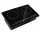During which time t does an electric cooker with power input P = 500 W and with efficiency n = 75% heat water with mass m = 2 kg and temperature t1 = 10°C to the boiling point (t2 = 100°C). The specific heat capacity of water is c = 4 180 J. Kg-1. K-1
• The tubIf we drop the 5 l from the first tap and the from second 2 liters of water, the water in the tub will have a temperature of 25°C. If we drop 3 l from the first tap and from second 4 l of water, the water in the tub will have a temperature of 21°C. Determ
• Bath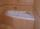In the bath is 30 liters of hot water. Then added 36 liters of cold water at temperature of 19 °C decreased temperature of water to 41 °C. What was the initial temperature of the hot water?
• PetraPetra likes to bathe in a bathtub with a water temperature of 38 degrees. She turned on the hot water set at 70 degrees and left for a moment. Before she returned, 40 liters of water flowed into the tub. How much water with a temperature of 22 degrees mus